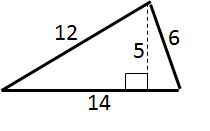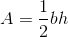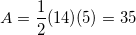# SSAT Middle Level Math : Plane Geometry

## Example Questions

← Previous 1 3 4 5 6 7 8 9 18 19

### Example Question #1 : Triangles

A right triangle has legs 90 centimeters and 16 centimeters, What is its area?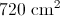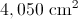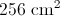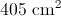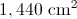Explanation:

The legs of a right triangle are its base and height, so use the area formula for a triangle with these dimension. Setting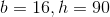: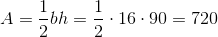### Example Question #2 : Triangles

A triangle has base 18 inches and height 14 inches. What is its area?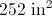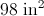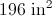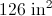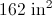Explanation:

Use the area formula for a triangle, setting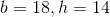: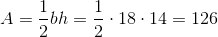### Example Question #3 : Triangles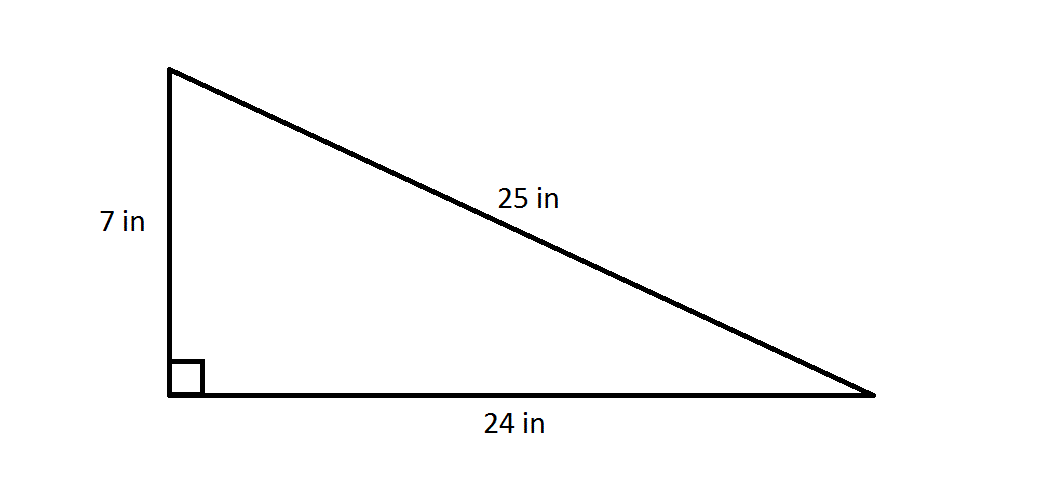What is the area of the above triangle?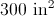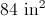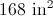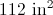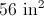Explanation:

The two legs of a right triangle can serve as its base and its height. The area of the triangle is half the product of the two: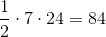That is, the area is 84 square inches.

### Example Question #4 : Triangles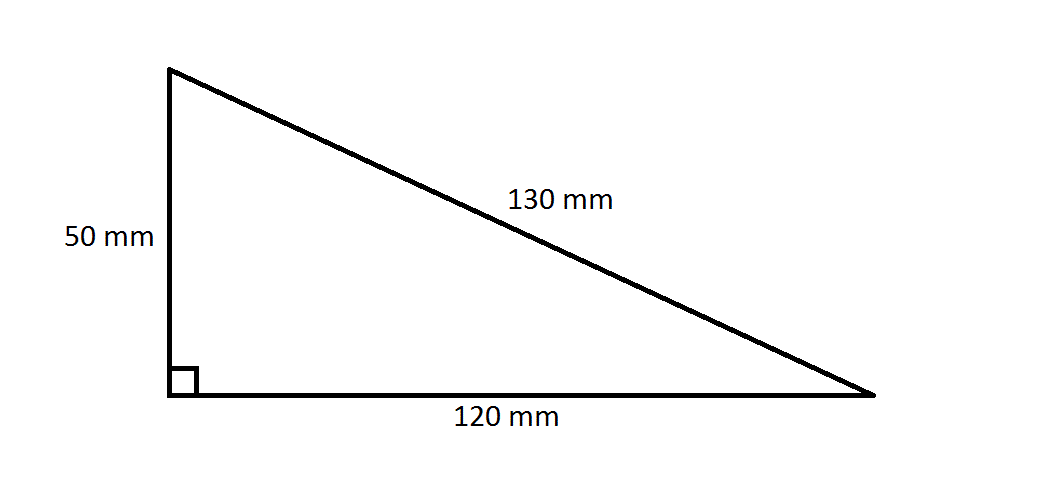What is the area of the above triangle?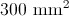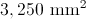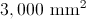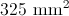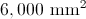Explanation:

The two legs of a right triangle can serve as its base and its height. The area of the triangle is half the product of the two: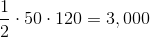That is, the area is 3,000 square millimeters.

### Example Question #5 : Triangles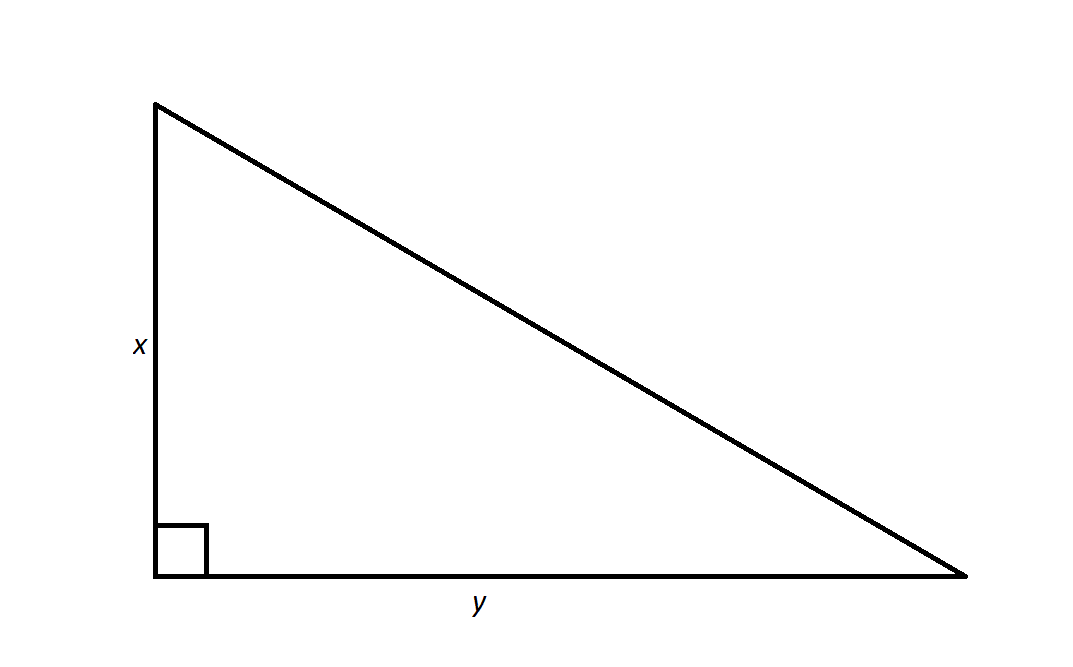Note: Figure NOT drawn to scale.

The above triangle has an area of 450 square centimers.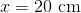. What is?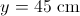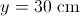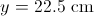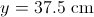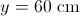Explanation:

The area of a triangle is one half the product of its base and its height - in the above diagram, that means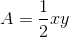.

Substitute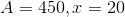, and solve for: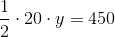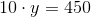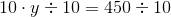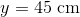### Example Question #6 : Triangles

Find the area of the triangle.

Note: Figure not drawn to scale.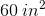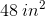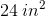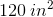Explanation:

To find the area of a triangle, multiply the base of the triangle by the height and then divide by two.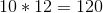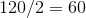### Example Question #7 : Triangles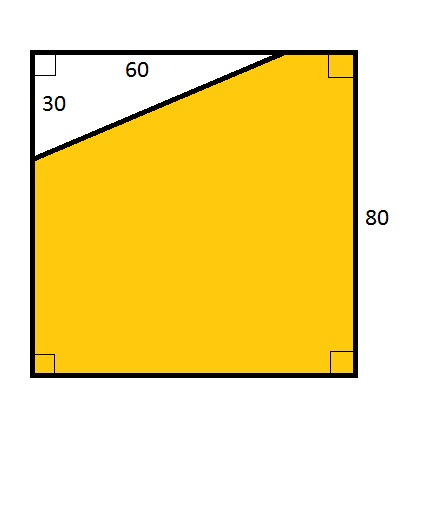The quadrilateral in the above diagram is a square. What percent of it is white?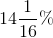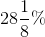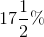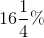Explanation:

The area of the entire square is the square of the length of a side, or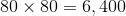.

The area of the white right triangle is half the product of its legs, or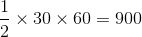.

Therefore, the area of that triangle is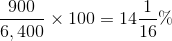of that of the entire square.

### Example Question #8 : Triangles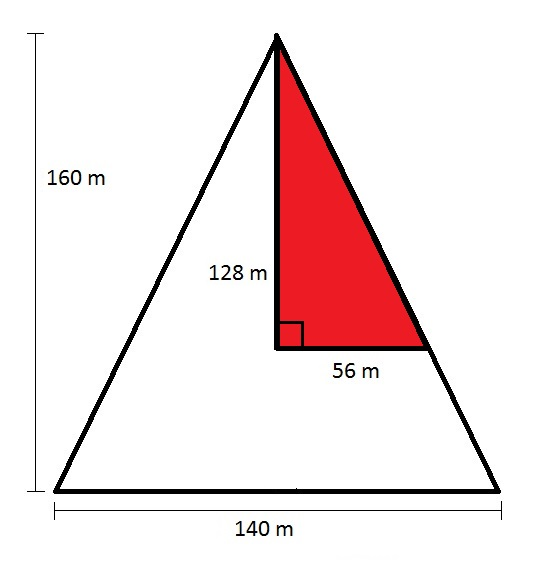Mr. Jones owns the isosceles-triangle-shaped parcel of land seen in the above diagram. He sells the parcel represented in red to his brother. What is the area of the land he retains?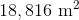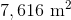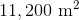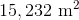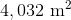Explanation:

The area of a triangle is half the product of its base and its height, so Mr. Jones's parcel originally had area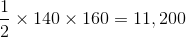square meters.

The portion he sold his brother, represented by the red right triangle, has area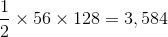square meters.

Therefore, the area of the parcel Mr. Jones retained is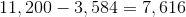square meters.

### Example Question #4 : Area Of A Triangle

Please use the following shape for the question.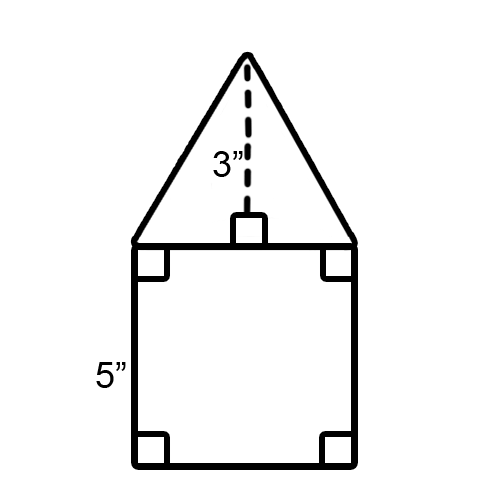What is the area of this shape?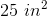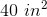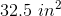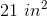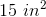Explanation:

From this shape we are able to see that we have a square and a triangle, so lets split it into the two shapes to solve the problem. We know we have a square based on the 90 degree angles placed in the four corners of our quadrilateral.

Since we know the first part of our shape is a square, to find the area of the square we just need to take the length and multiply it by the width. Squares have equilateral sides so we just take 5 times 5, which gives us 25 inches squared.

We now know the area of the square portion of our shape. Next we need to find the area of our right triangle. Since we know that the shape below the triangle is square, we are able to know the base of the triangle as being 5 inches, because that base is a part of the square's side.

To find the area of the triangle we must take the base, which in this case is 5 inches, and multipy it by the height, then divide by 2. The height is 3 inches, so 5 times 3 is 15. Then, 15 divided by 2 is 7.5.

We now know both the area of the square and the triangle portions of our shape. The square is 25 inches squared and the triangle is 7.5 inches squared. All that is remaining is to added the areas to find the total area. Doing this gives us 32.5 inches squared.

### Example Question #1 : Area Of A Triangle

What is the area of the triangle?